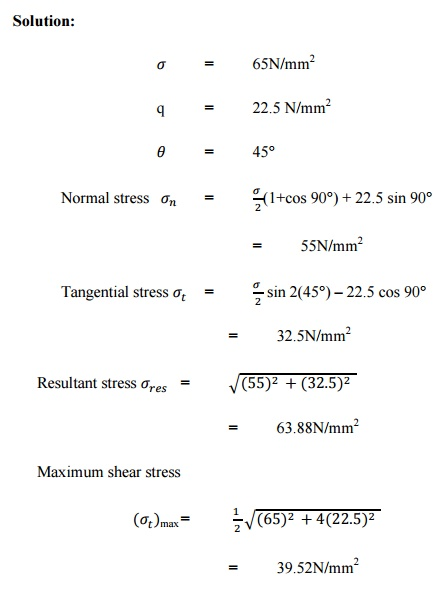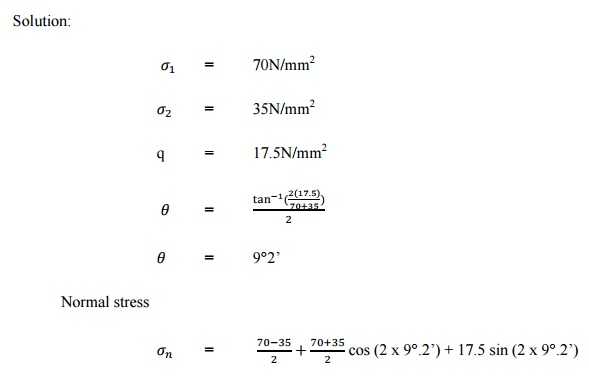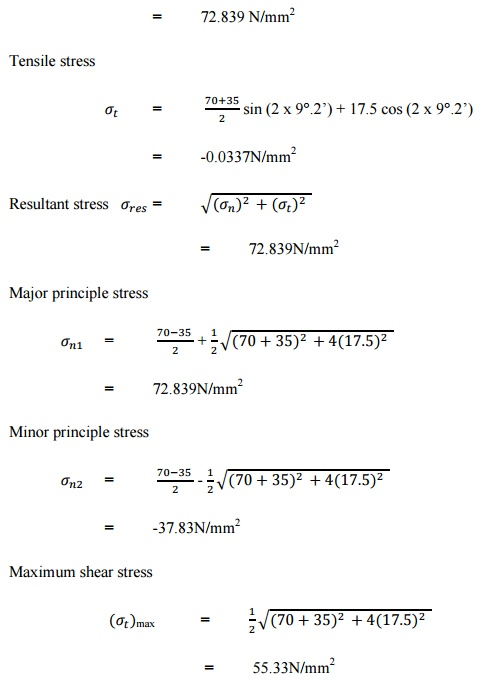Home | | Strength of Materials for Mechanical Engineers | Solved Problems: Thin Cylinders, Spheres and Thick Cylinders

# Solved Problems: Thin Cylinders, Spheres and Thick Cylinders

Mechanical - Strength of Materials - Thin Cylinders, Spheres and Thick Cylinders

Problem -1:

The mild steel block has cross-section of 50x50mm carries an axial load of 35KN which is compressive in nature. Find the normal, tangential stresses across the plane through the point of 30 to the axis of the block. Also find the maximum shear stress in the block. (Apr/May 2008)

Solution:Problem -2:

A member subjected to a pull of two pieces wooden frame of cross section (35x15)mm connected by bolts joints. Calculate the maximum permissible value of P which can withstand if the permissible normal, tangential stresses is 13N/mm2 and 8N/mm2. Angle of cross section is 40 .

Solution:Problem-3:

A 5mm thick aluminium plate has a width of 300mm and a length of 600mm subjected to pull of 15000N,9000N respectively in axial transverse directions. Determine the normal, tangential and resultant stresses on a plate 50Â°.Problem â€“4:

The principle stresses at a point in the section of a heat exchanger shell are 18MPa (Tensile) and 10MPa (Compressive). Acting mutually perpendicular to each other. Determine the normal, shear, resultant stress on a plane whase normal is inclilned at 60Â° to 10MPa stress. Find also the maximum shear stress.

Solution:Problem -5:

A steel bar is under a tensile stress of 65N/mm2 at the same time it is accompanied by a shear stress of 22.5N/mm2. Find the normal, shear stress and resultant stress across a plane at an angle of 45Â° with the axis of major tensile stress and also find the maximum shear stress.

Solution:Problem -6:

A rectangular block of material is subjected to a tensile stress of 110N/mm2, one plate and a tensile stress of 47N/mm2 on another plate at right angle to each other and the above stress is accomplished by a shear stress of 63N/mm2. Determine the principle stress, principle plane and maximum shear stress.

Solution:Problem -7:

Two planes AB,AC, which are right angles carries a shear stress of intensity 17.5N/mm2 while this plane also carrying a tensile stress of 70N/mm2 at a compressive stress of 35N/mm2 respectively. Determine the normal tangential & resultant stress, principle planes and principle stress and also determine the maximum shear stress.

Solution:Study Material, Lecturing Notes, Assignment, Reference, Wiki description explanation, brief detail
Mechanical : Strength of Materials : Thin Cylinders, Spheres and Thick Cylinders : Solved Problems: Thin Cylinders, Spheres and Thick Cylinders |